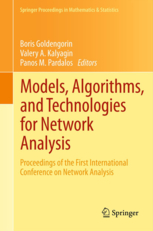• A
• A
• A
• ABC
• ABC
• ABC
• А
• А
• А
• А
• А
Regular version of the site

## Pattern-Based Heuristic for the Cell Formation Problem in Group Technology

P. 11-50.
Mikhail Batsyn, Ilya Bychkov, Boris Goldengorin, Panos M. Pardalos, Pavel Sukhov.

In this paper we introduce a new pattern-based approach within the Linear Assignment Model with the purpose to design heuristics for a combinatorial optimization problem (COP). We assume that the COP has an additive (separable) objective function and the structure of a feasible (optimal) solution to the COP is predefined by a collection of cells (positions) in an input file. We define a pattern as a collection of positions in an instance problem represented by its input file (matrix). We illustrate the notion of pattern by means of some well known problems in COP among them the Linear Ordering Problem, Cell Formation Problem (CFP) just to mention a couple. The CFP is defined on a Boolean input matrix which rows represent machines and columns - parts. The CFP consists in finding three optimal objects: a block-diagonal collection of rectangles, a rows (machines) permutation, and a columns (parts) permutation such that the grouping efficacy is maximized. The suggested heuristic combines two procedures: the pattern-based procedure to build an initial solution and an improvement procedure to obtain a final solution with high grouping efficacy for the CFP. Our computational experiments with the most popular set of 35 benchmark instances show that our heuristic outperforms all well known heuristics and returns either the best known or improved solutions to the CFP.

### In bookIss. 32. NY: Springer, 2013.